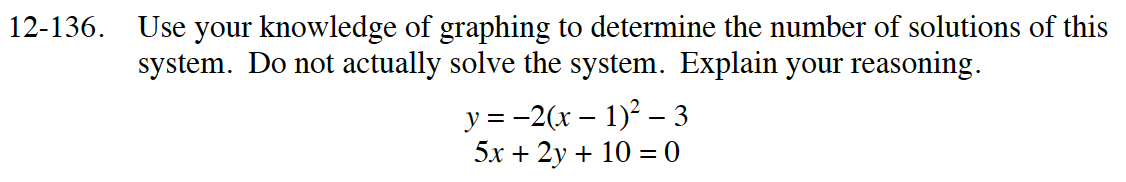### Home > INT2 > Chapter Ch12 > Lesson 12.2.4 > Problem12-136

12-136.
1. Use your knowledge of graphing to determine the number of solutions of this system. Do not actually solve the system. Explain your reasoning. Homework Help ✎

2. y = −2(x − 1)2 − 3
5x + 2y + 10 = 0Rewrite the equation of the line in y = mx + b form.
Think about where would it be located on a graph.

Where is the vertex of the parabola?
Which direction does it open?